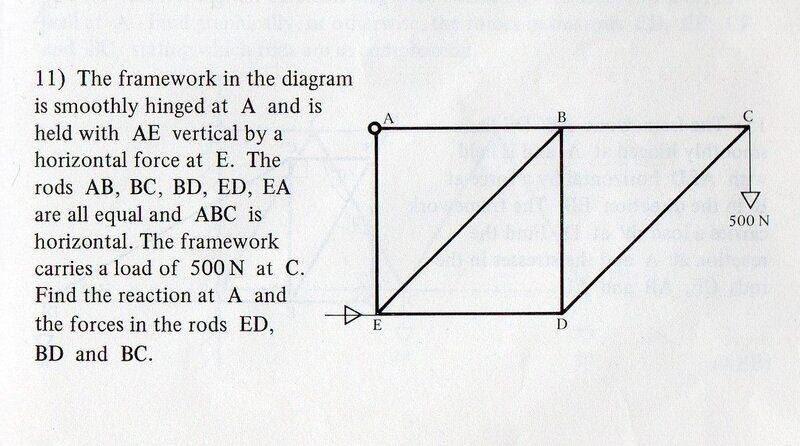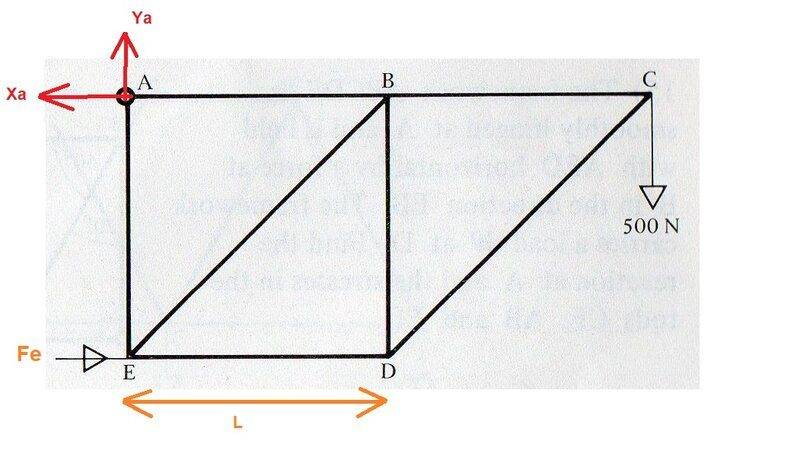# To find forces in a framework

gnits
Homework Statement:
To find forces in a framework
Relevant Equations:
Equating of forces
Moments
A very simple (I thought!) question:I'm just looking at the first part, finding the reaction at the hinge A.

Here is my annotated diagram, with the reaction and A resolved into it's X and Y components, the force at E labelled as Fe and the length of ED labelled as L.Considering the body as a whole:

Resolving vertically:

Ya = 500

Xa * L = 2 * L * 500

which gives Xa = 1000

So the resultant at A will have size sqrt(1000^2 + 500^2) = 1118 (approx.)

Answer given in book is 112 N at 26.6⁰ below the horizontal.

My answer is very different and also above the horizontal.

What simple mistake have I made in my reasoning?

Thanks.

Homework Helper
Gold Member
The answer given in the book is not correct, yours is.

gnits
Thanks, that's a relief. The book has rarely been wrong and so I was all too ready to doubt myself. Much appreciated.

•Lnewqban